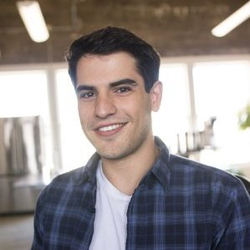# Car Detection for Autonomous Driving

Let us take a look at object detection using the very powerful YOLO model. Many of the ideas here are described in the two YOLO papers: Redmon et al., 2016 (https://arxiv.org/abs/1506.02640) and Redmon and Farhadi, 2016 (https://arxiv.org/abs/1612.08242).

YOLO (“you only look once”) is a popular algoritm because it achieves high accuracy while also being able to run in real-time. This algorithm “only looks once” at the image in the sense that it requires only one forward propagation pass through the network to make predictions. After non-max suppression, it then outputs recognized objects together with the bounding boxes.

Here’s one way to visualize what YOLO is predicting on an image. For each of the 19x19 grid cells, we can find the maximum of the probability scores (taking a max across both the 5 anchor boxes and across different classes). I we color that grid cell according to what object the grid cell considers the most likely, we get the following result.

Note that this visualization isn’t a core part of the YOLO algorithm itself for making predictions; it’s just a nice way of visualizing an intermediate result of the algorithm.

Another way to visualize YOLO’s output is to plot the bounding boxes that it outputs. Doing that results in a visualization like this.

In the figure above though, we plotted only boxes that the model had assigned a high probability to and this is still too many boxes. We’d like to filter the algorithm’s output down to a much smaller number of detected objects. To do so, we’ll use non-max suppression. Specifically, we’ll carry out these steps:

• Get rid of boxes with a low score, i.e. box is not very confident about detecting a class
• Select only one box when several boxes overlap with each other and detect the same object

Even after filtering by thresholding over the classes scores, we still end up a lot of overlapping boxes. A second filter for selecting the right boxes is called non-maximum suppression (NMS).

Applying the YOLO model to a hold-out image, an example result is the following.##### Eric M. Fischer
###### Ph.D. Statistics with specialization in Artificial Intelligence

My research interests are in natural language processing and generative modeling.